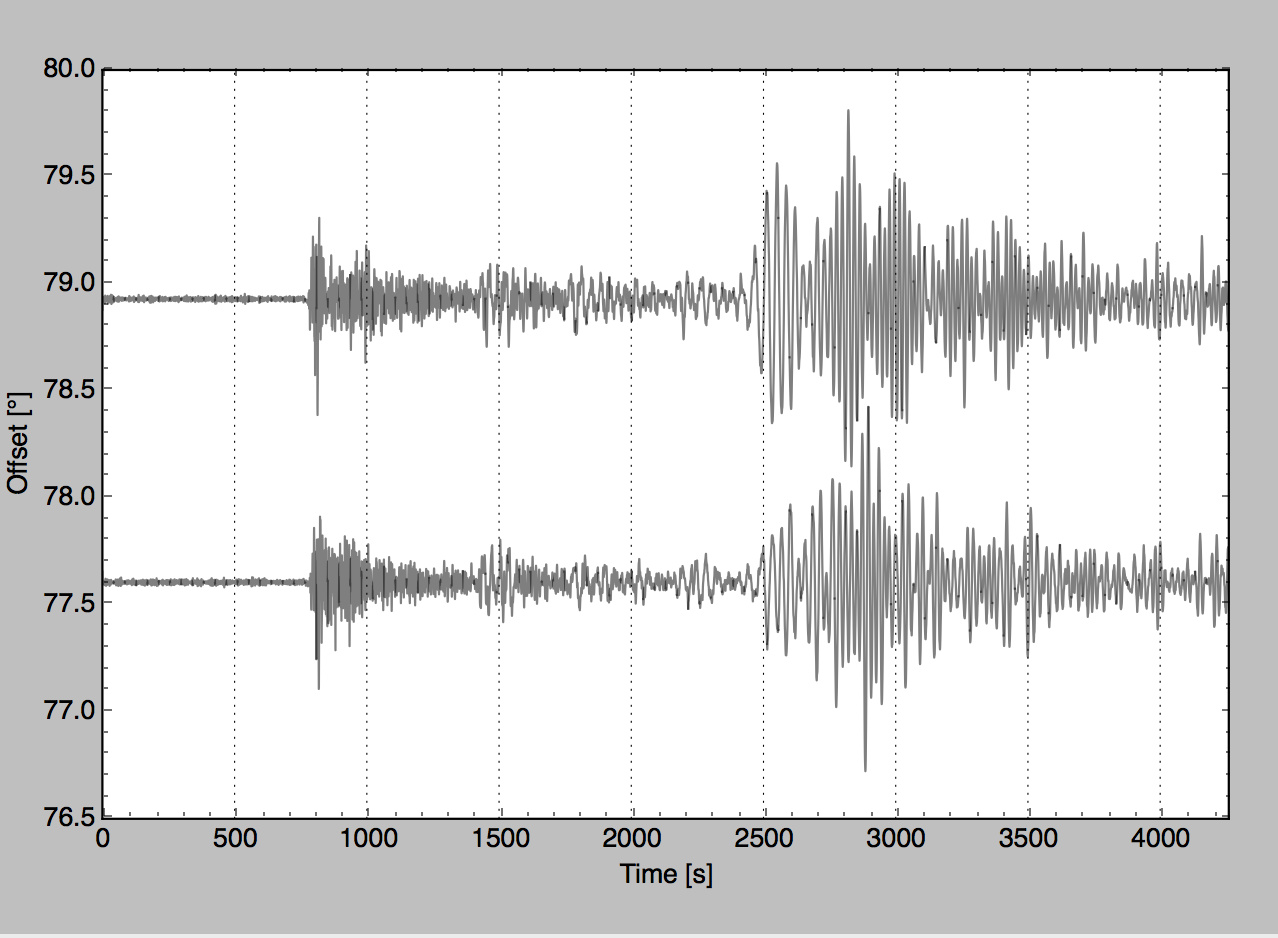# Obspy section plot labels

Hi all,

I am trying to write station name label on a horizontal section plot with distance in degrees using the function here under:

st.plot(fig=fig, type='section', dist_degree=True, scale=2, orientation='horizontal', ev_coord=(evla, evlo))

Here is the plot. I would like to add the station name labels at the right side of the plot, closed to each plot.

I don't know how to do it. Do you have an idea?

Regards,
Coralie